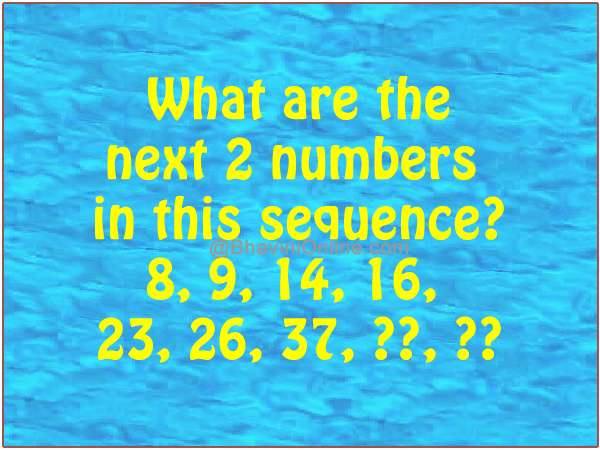# What Are The Next 2 Numbers In This Sequence: 8, 9, 14, 16, 23, 26, 37

Challenge your brain with this tough number series riddle.

What are the next 2 numbers in this sequence?

8, 9, 14, 16, 23, 26, 37Share it with your friends to test their IQ…

There are actually 2 alternate series in the sequence.

The first series is (+1, +2, +3, +4…) &

the second series is (+5, +7, +11, +13….) (Prime numbers)

8 + 1 = 9;

9 + 5 = 14;

14 + 2 = 16;

16 + 7 = 23;

23 + 3 = 26;

26 + 11 = 37;

37 + 4 = 41;

41 + 13 = 54.

So the number are 41, 54